# 进制转换

### 目录

`进制`：简单来说，就是一种进位的方式。x进制，表示逢x进1。
`二进制`：只由0，1组成的数据就是二进制数据 例如：10110110
`八进制`：把二进制的数据，从右开始，每三位一组合，最左边不够的时候，补0。然后，分别计算出对应的十进制数值，最后，在把每个十进制的数据组合起来，就是一个八进制的数据。将二进制数据`10110110`转换为八进制数据，如图所示：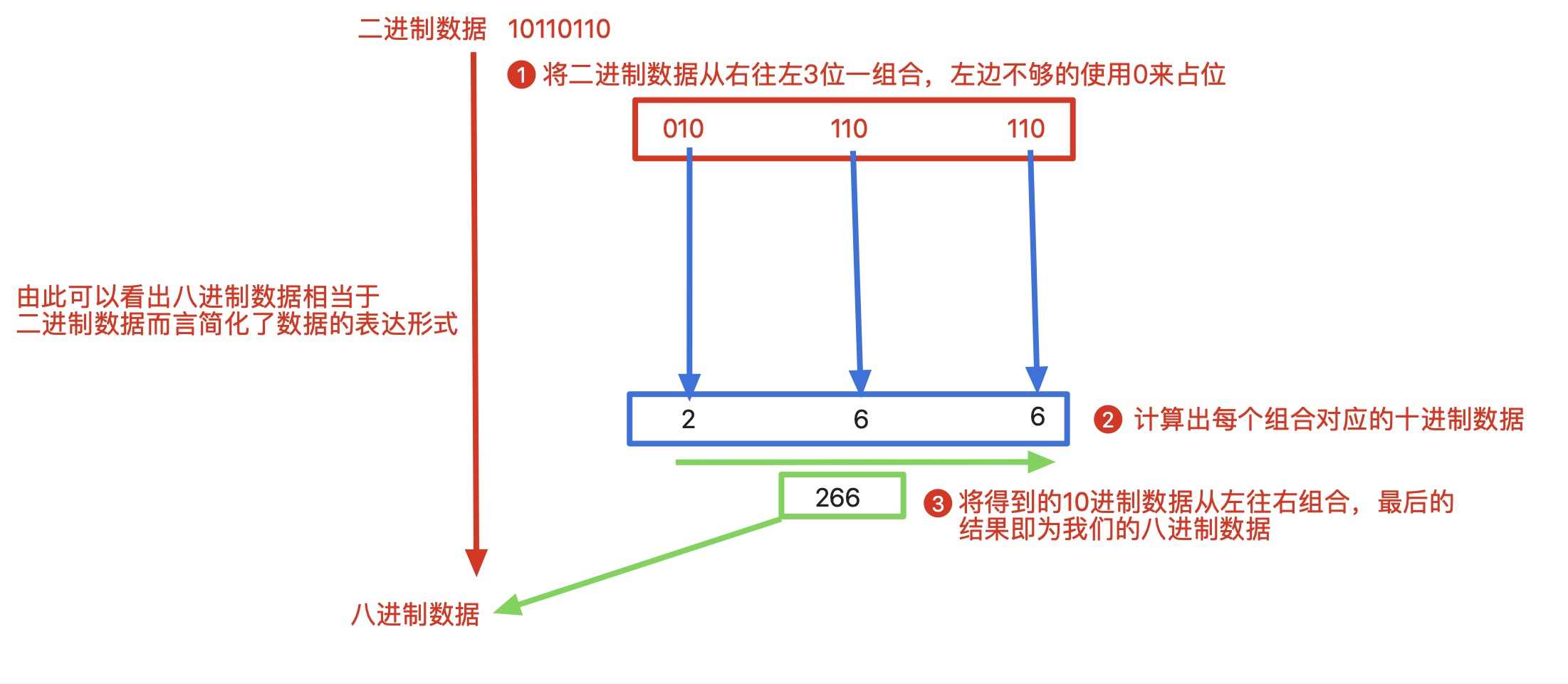`十六进制`：上面表现的形式还不是最简单的，我们还有更简单的。把二进制的数据，从右开始，每四位一组合，最左边不够的时候，补0。然后，分别计算出对应的十进制数值，最后，在把每个十进制的数据组合起来，就是一个十六进制数据。将二进制数据`10110110`转换为十六进制数据，如图所示：`规则：进制越大，表现形式越短。`

# 1. 任意进制到十进制的转换

``````十进制                                   十进制
12345 = 10000 + 2000 + 300 + 40 + 5
= 1 * 10^4  + 2 * 10^3 + 3 * 10^2 + 4 * 10^1 + 5 * 10^0
= 10000 + 2000 + 300 + 40 + 5
= 12345
``````

`系数`：每一个位上的数据值本身就是系数。
`基数`：x进制的基数就是x。
`权`：我们针对每一个位上的数据进行编号，从右边，并且是从0开始编号，这个编号就是该位值上数据的权值。
`结论`：十进制数据 = 系数 * 基数^权次幂相加。

`二进制`数据转换为`十进制`数据，如下所示：

``````十进制数据 = 二进制数据每个位置上的数据值 * 2^权次幂相加

100 = 1 * 2^2 + 0 * 2^1 + 0 * 2^0
= 4 + 0 + 0
= 4
``````

`八进制`数据转换为`十进制`数据，如下所示：

``````十进制数据 = 八进制数据每个位置上的数据值 * 8^权次幂相加

100 = 1 * 8^2 + 0 * 8^1 + 0 * 8^0
= 64 + 0 + 0
= 64
``````

`十六进制`数据转换为`十进制`数据，如下所示：

``````十进制数据 = 十六进制数据每个位置上的数据值 * 16^权次幂相加

100 = 1 * 16^2 + 0 * 16^1 + 0 * 16^0
= 256 + 0 + 0
= 256
``````

# 2. 十进制到任意进制的转换

`十进制`数据`20`转换为`二进制`数据`10100`，如图所示：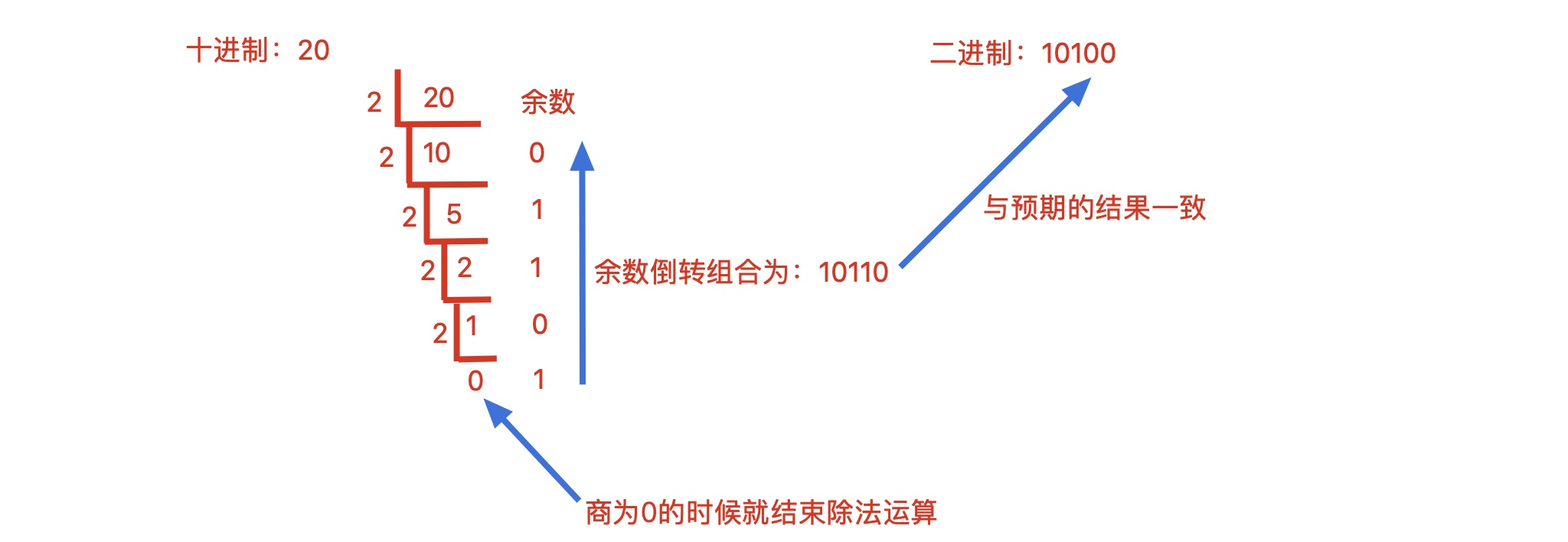`十进制`数据`20`转换为`八进制`数据`24`，如图所示：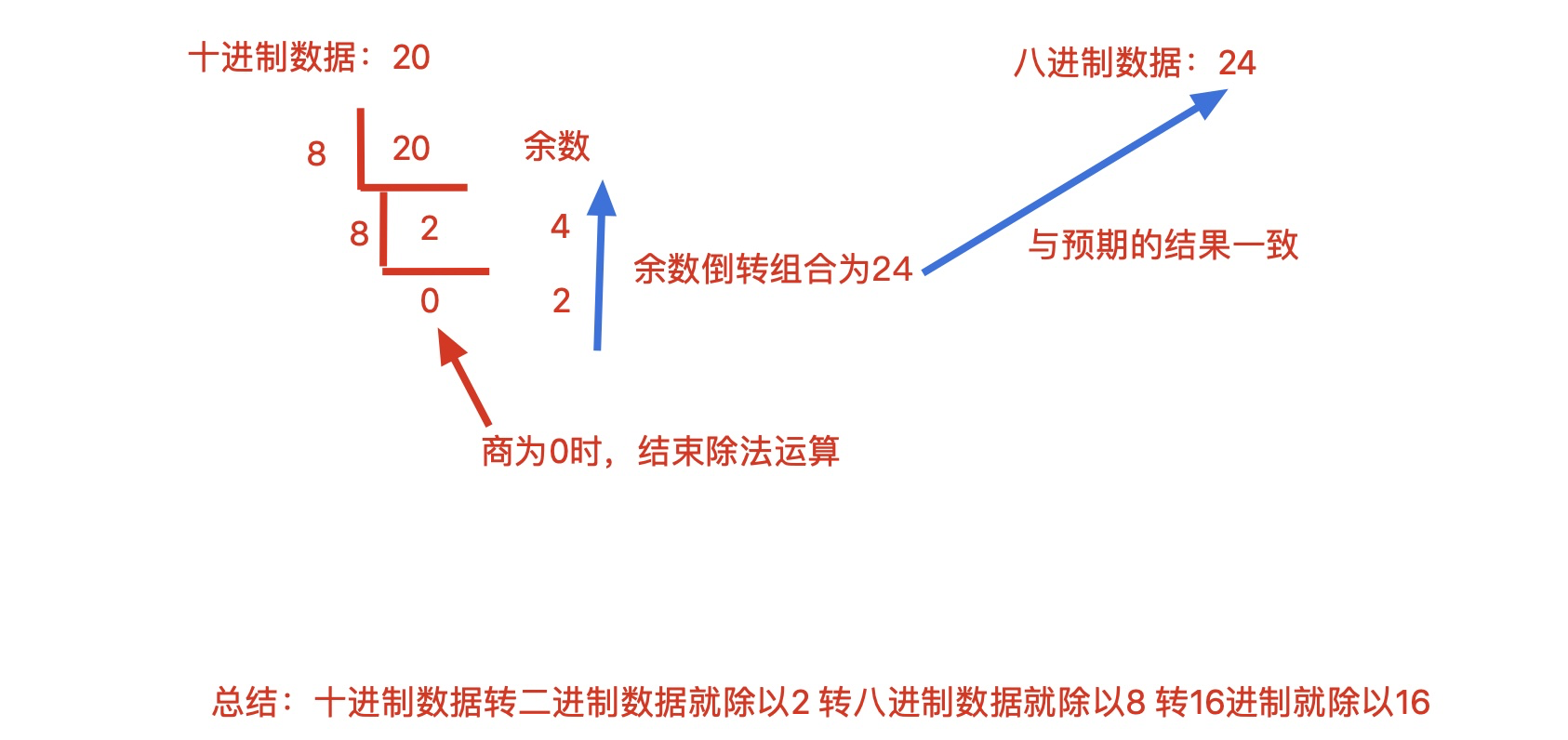`十进制`数据`20`转换为`十六进制`数据`14`，如图所示：# 3. 快速的进制转换法

`8421码`，是`bcd`码的一种。它表达的意思是每一个二进制位上的数据对应一个固定的值，只需要把对应的1位置的数据值给相加，即可得到该二进制对应的十进制的值。如图所示：``````二进制到十进制的转换：
1010100 =  64 + 16 + 4 = 84

100 = 64 + 32 + 4 = 0b1100100
``````

``````x进制   -->  十进制

``````

``````A: 二进制到十进制，十进制到八或者十六进制
B: 拆分组合法
``````# 4. Python中进制转换函数

1. 十进制整数：十进制整数的表现形式大家都很熟悉。例如，下面的数值都是有效的十进制整数。
``````num1 = 10000
num2 = 256  # 注意：不能以0作为十进制数的开头（0除外）。
``````
1. 八进制整数：由0~7组成，进位规则为`逢八进一`，并且以`0o/0O`开头的数，如`0o123`(转换成十进制数为83)、-0o123（转换成十进制数为-83）。注意: `0o/0O`只是一个标示符号，真正参与转换的是后面的数据。在`Python 3.x`中，八进制数，必须以`0o/0O`开头。但在`Python 2.x`中，八进制数可以以`0`开头。
2. 十六进制整数：由`0~9``A~F`组成，进位规则为`逢十六进一`，并且以`0x/0X`开头的数，如`0x25`（转换成十进制数为37）、`0Xb01e`（转换成十进制数为45086）。
3. 二进制整数：由0和1两个数组成，进位规则是`逢二进一`，并且以`0b/0B`开头的数,如`0b101`（转换成十制数后为5）、`0b1010`（转换成十进制数后为10）。

## 4.1 bin()函数

bin()函数用于将整数转换为前缀以`0b`开头的二进制字符串形式，bin()函数的语法格式如下：

``````bin(x)  # x：要转换的整数
# 返回值: 返回以0b开头的二进制字符串
``````

``````number = int(input('输入一个十进制数：'))  # 输入用户需要转换的数字
print(bin(number))  # 输出对应的二进制数
``````## 4.2 hex()函数

hex()函数用于将整数转换为以`0x`开头的十六进制字符串形式。hex()函数的语法格式如下：

``````hex(x)  # 参数x：要转换的整数
# 返回值: 返回一个以0x开头的十六进制字符串
``````

``````print(hex(2))
print(hex(8))
print(hex(19))
print(hex(-88))
print(hex(250))
``````## 4.3 oct()函数

oct()函数用于将整数转换为前缀以`0o`开头的八进制字符串形式，oct()函数的语法格式如下：

``````oct(x)  # x：要转换的整数
# 返回值: 返回前缀以0o开头的八进制字符串形式。
``````

``````# 输出对应的八进制字符串形式
print(oct(50))
print(oct(45))
print(oct(-33))
``````## 4.4 int()函数

int()函数可用来把浮点数转换为整数，或者把字符串按指定进制数转换为整数。int()函数的语法格式如下：

``````int(x [,base])
``````

1. x：数字或者字符串；
2. base：表示进制数，默认值为10，即默认为十进制数，用中括号括起来，意思是可以省略；
3. 返回值：返回整数；int()函数不提供任何参数时，返回的结果为0。如图所示：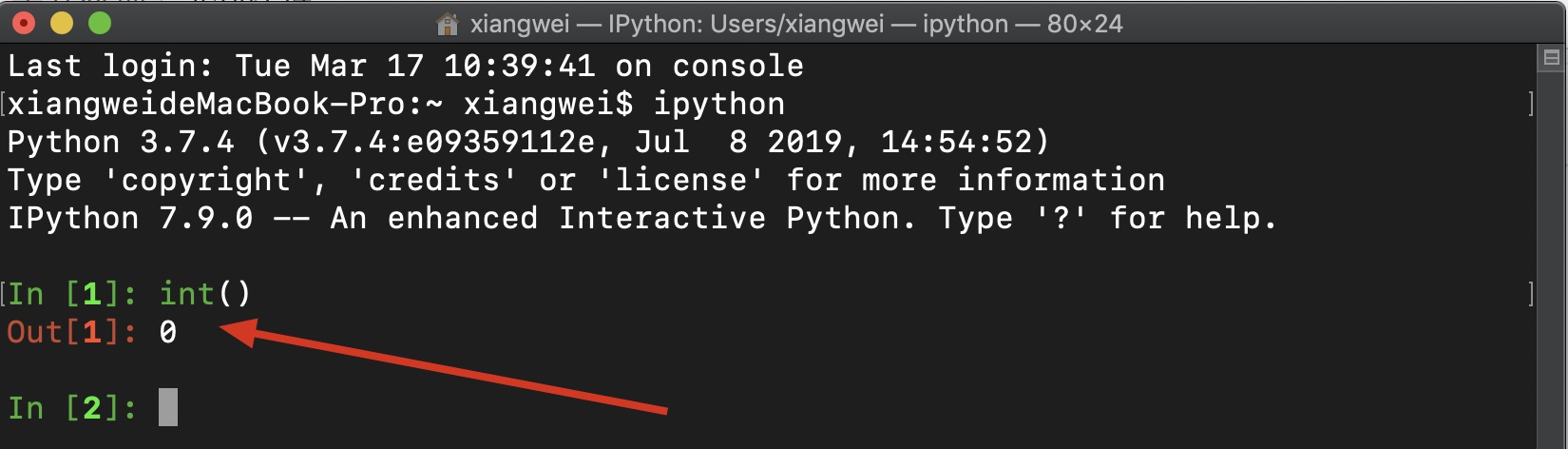注意：int()函数中参数x可以是数字或字符串，但是参数base被赋值后，参数x只能是字符串，否则会提示`int() can't convert non-string with explicit base`错误（当参数base存在时，int()函数不能转换非字符串类型）。如图所示：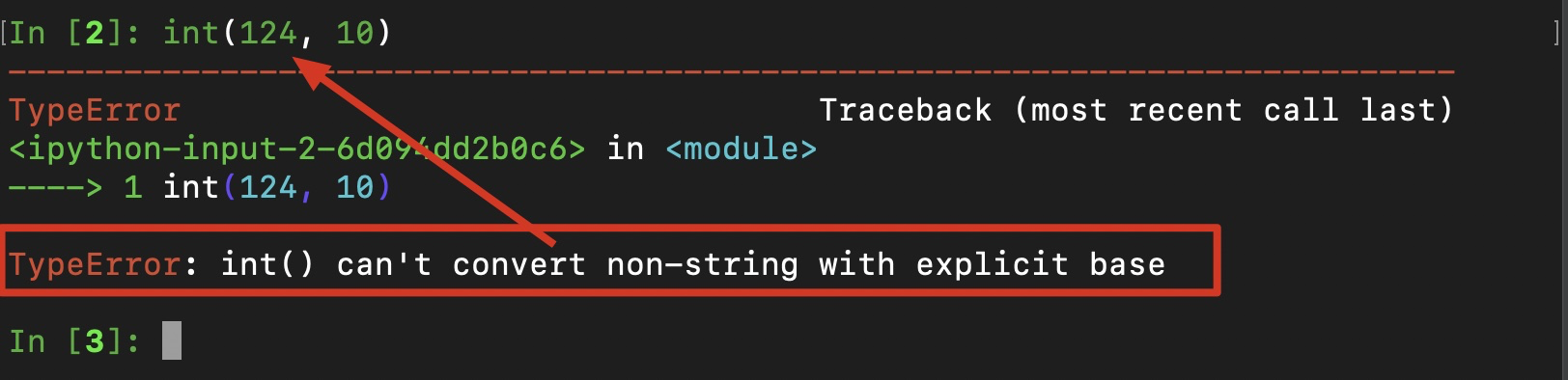如果int()函数中参数x为浮点数，则只取整数部分。如图所示：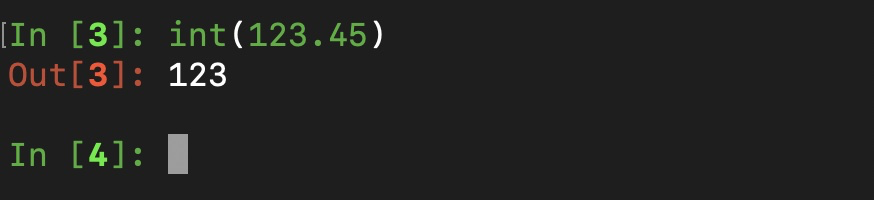参数为字符串时，并指定了进制数，则按对应进制数将字符串转换成十进制整数，如图所示：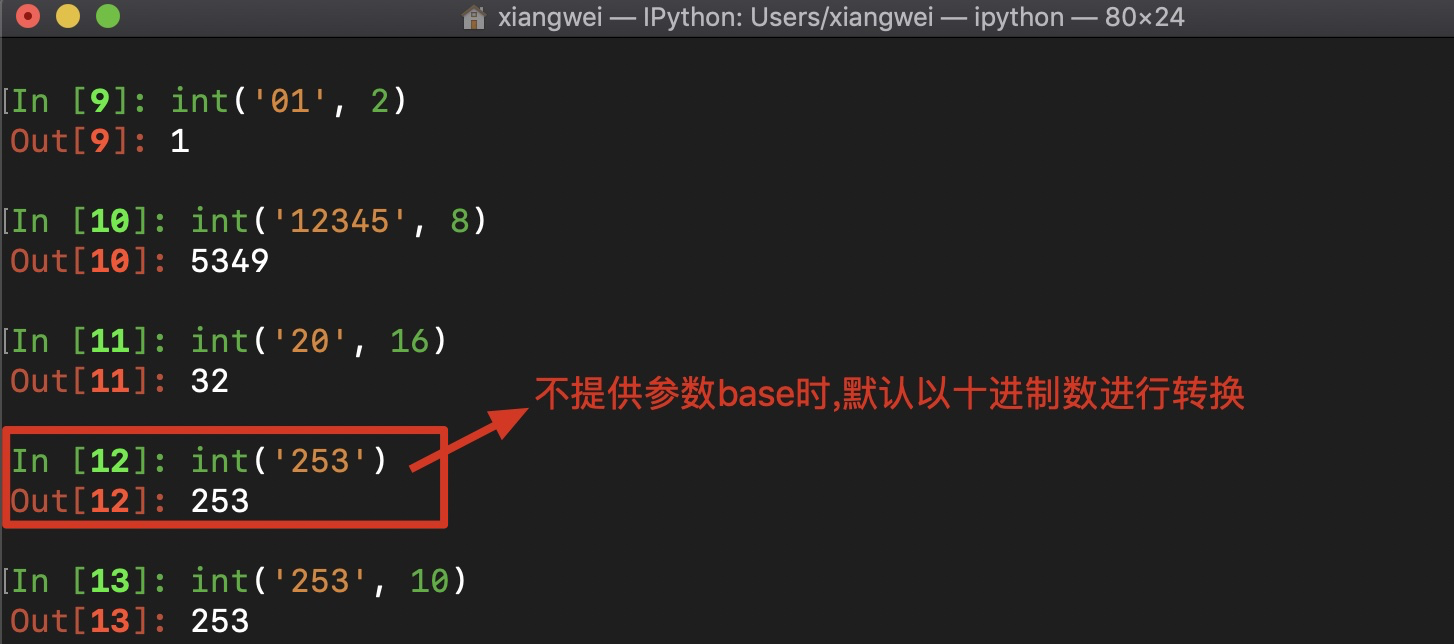int函数还有一个奇葩的地方，通过input输入小数时（如5.20），使用int函数将其转换为整数时出现错误，如图所示：经研究发现在input中将小数转为整数，需要先使用float函数将其转为浮点型，然后再使用int函数转为整型，代码如下：
``````my_val = input('请输入小数:')
print(type(int(float(my_val))))
``````

运行结果，如图所示：